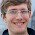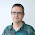## Monday, July 7, 2014

### Using the D3 trail layout to draw the Hayian tracks

I wrote many examples (1, 2, 3 and 4) and some entries in the blog (1 and 2) showing how to draw animated paths on a map using the D3 library.
But since then, Benjamin Schmidt wrote a D3 layout, called trail layout, that simplifies a lot doing this kind of stuff.
Since the layout is new, and hasn't got many examples (actually, two made by the author), I'll try to show how to work with it.

### The trail layout

How does the trail layout work? The author defines it as:
This is a layout function for creating paths in D3 where (unlike the native d3.svg.line() element) you need to apply specific aesthetics to each element of the line.
Basically, the input is a set of points, and the layout takes them and creates separate segments to join them. This segments can be either line or d SVG elements.

#### Let's see the simplest example:



var width = 600,
height = 500;

var points = [{"x":0,"y":0}, {"x":200,"y":200}, {"x":0,"y":400}, {"x":200,"y":100}];
var svg = d3.select("body").append("svg")
.attr("width", width)
.attr("height", height);

var trail = d3.layout.trail().coordType('xy');

var trail_layout = trail.data(points).layout();

paths = svg.selectAll("line").data(trail_layout);

paths.enter()
.append('line')
.style("stroke-width",3)
.style("stroke","black")
.attr("x1",function(d) {return d.x1})
.attr("y1",function(d) {return d.y1})
.attr("y2",function(d) {return d.y2})
.attr("x2",function(d) {return d.x2})

• In this case, the points are defined as an array of objects with the x and y properties. If the x and y are named this way, the layout takes them directly. If they are called for instance lon and lat, the layout must be told how to get them.
• Line 10 creates the SVG
• Line 14 initializes the layout. In this case, the layout is using the coordType xy, which means that as a result will give the initial and end point for each segment, convenient for drawing SVG line elements. The other option is using the coordinates value, which is convenient for drawing d elements, as we will see later.
•  Line 15 is where the data is set and the layout is retrieved
• The last step is where the lines are actually drawn.
• For each data element, the line svg is added
• The styles are applied
• The extremes of the line are set using the attributes x1, y1, x2, y2

#### How to use coordinates as the coordType:

The following example created the trail as a set of SVG line elements, but the trail layout has an option for creating it as a set of SVG d elements (paths).
You can see the example here. The data, in this case, is the Hayian track. As you can see, it's quite similar as the former example, with the following differences:
• Since in this case we are using geographical coordinates, a projection must be set, and also a d3.geo.path to convert the data into x and y positions, as usual when drawing d3 maps
• When initializing the trail layout, coordinates must be set as the coordType.
• Since the data elements do not store the positions with the name x and y, the layout has to be told how the retrieve them using the positioner:
.positioner(function(d) {return [d.lon, d.lat];})
• When drawing the trail, a path element is appended instead the line element, and the d attribute is set with the path  function defined above.

### Creating the map with the trail

Once the basic usage of the trail layout is known, let's reproduce the Hayian path example (simplified for better understanding):


.map {
fill: none;
stroke: #777;
stroke-opacity: .5;
stroke-width: .5px;
}

.land {
fill: #999;
}

.boundary {
fill: none;
stroke: #fff;
stroke-width: .5px;
}

var width = 600,
height = 500;

var projection = d3.geo.mercator()
.scale(5*(width + 1) / 2 / Math.PI)
.translate([width / 2, height / 2])
.rotate([-125, -15, 0])
.precision(.1);

var path = d3.geo.path()
.projection(projection);

d3.json("/mbostock/raw/4090846/world-50m.json", function(error, world) {
d3.json("track.json", function(error, track) {

var color_scale = d3.scale.quantile().domain([1, 5]).range(colorbrewer.YlOrRd);

var svg = d3.select("body").append("svg")
.attr("width", width)
.attr("height", height);

var trail = d3.layout.trail()
.positioner(function(d) {return projection([d.lon,d.lat]);})
.coordType('xy');

var trail_layout = trail.data(track).layout();

svg.insert("path", ".map")
.datum(topojson.feature(world, world.objects.land))
.attr("class", "land")
.attr("d", path);

svg.insert("path", ".map")
.datum(topojson.mesh(world, world.objects.countries, function(a, b) { return a !== b; }))
.attr("class", "boundary")
.attr("d", path);

var hayan_trail = svg.selectAll("d").data(trail_layout);

hayan_trail.enter()
.append('line')
.attr("x1",function(d) {return d.x1})
.attr("x2",function(d) {return d.x1})
.attr("y1",function(d) {return d.y1})
.attr("y2",function(d) {return d.y1})
.attr("class","line")
.style("stroke-width",4)
.attr("stroke", function(d){return color_scale(d.class);})
.transition()
.ease("linear")
.delay(function(d,i) {return i*500})
.duration(500)
.attr("x2",function(d) {return d.x2})
.attr("y2",function(d) {return d.y2})
;

});
});

• The map creation is as usual (explained here)
• Lines 49 to 51 create the trail layout as in the former example
• Line 67 creates the trail, but with some differences:
• the beginning and the end of the line are the same point at the beginning, so the line is not drawn at this moment (lines 69 to 72)
• The stroke colour is defined as a function of the typhoon class using the colour scale (line 75)
• A transition is defined to create the effect of the line drawing slowly
• The ease is defined as linear, important in this case where we join a transition for each segment.
• The delay is set to draw one segment after the other. The time (500 ms) must be the same as the one set at duration
• Finally, the changed values are x2 and y2, that is, the final point of the line, which are changed to their actual values
• The complete example, with the typhoon icon and the date is also available
It's possible to use paths instead of lines to draw the map, as in the first version. The whole code is here, but the main changes are in the last section:
hayan_trail.enter()
.append('path')
.attr("d", path)
.style("stroke-width",7)
.attr("stroke", function(d){return color_scale(d.class);})
.style('stroke-dasharray', function(d) {
var node = d3.select(this).node();
if (node.hasAttribute("d")){
var l = d3.select(this).node().getTotalLength();
return l + 'px, ' + l + 'px';
}
})
.style('stroke-dashoffset', function(d) {
var node = d3.select(this).node();
if (node.hasAttribute("d"))
return d3.select(this).node().getTotalLength() + 'px';
})
.transition()
.delay(function(d,i) {return i*1000})
.duration(1000)
.ease("linear")
.style('stroke-dashoffset', function(d) {
return '0px';
});
• The strategy here is to change the stroke-dasharray  and stroke-dashoffset style values as in this example, and changing it later so the effect takes place.
• At the beginning, both values are the same length as the path. This way, the path doesn't appear. The length is calculated using the JavaScript function getTotalLength
• After the transition, the stroke-offset value will be 0, and the path is fully drawn

### Conclusion

I recommend using the trail layout instead of the method from my old posts. It's much cleaner, fast, easy, and let's changing each segment separately.
The only problem I find is that when the stroke width gets thicker, the angles of every segment make strange effects, because the union between them doesn't exist.

This didn't happen with the old method. I can't imagine how to avoid this using lines, but using the coordinates option could be solved transforming the straight lines for curved lines.

1.1.